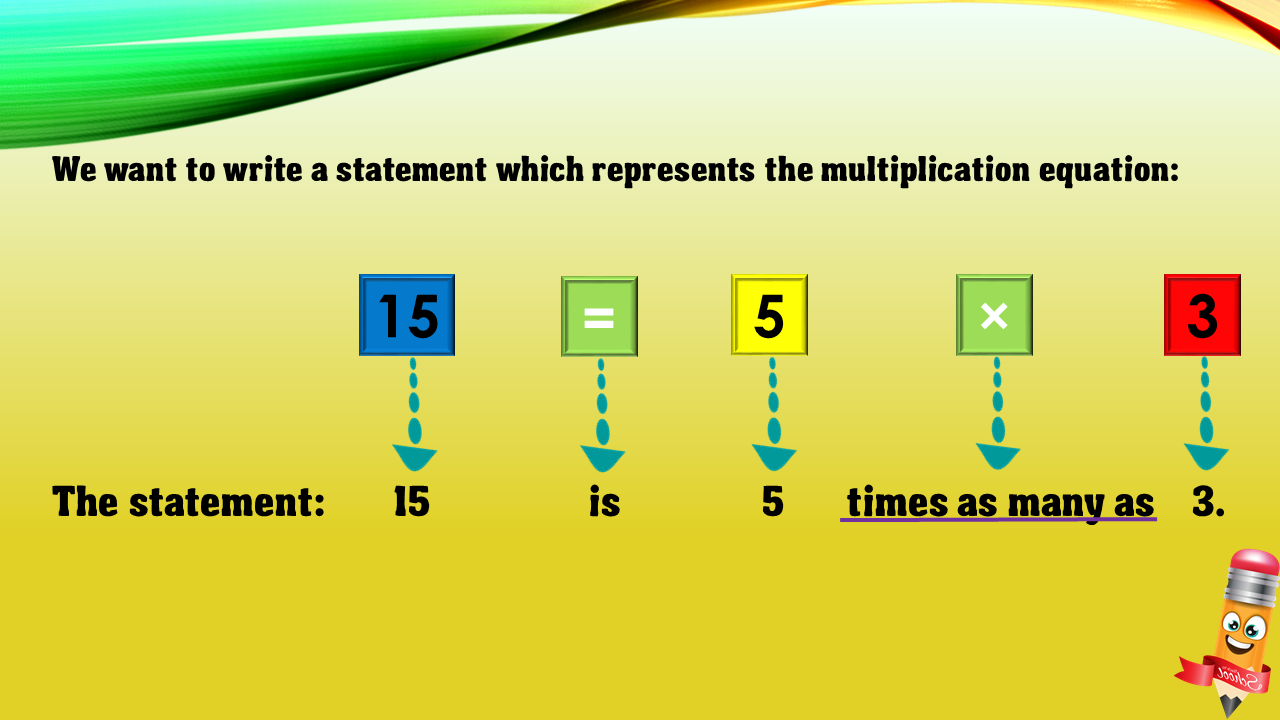1
visibility

Choose one statement which represents the equation: 15 = 5×3 ?

• A

15 times as many as 5 is 3.

• B

15 is 5 times as many as 3.

• C

15 is 5 more than 3.

• D

5 is 3 less than 15.